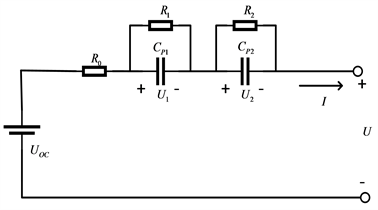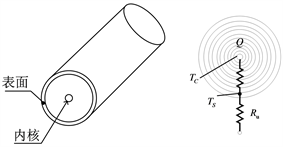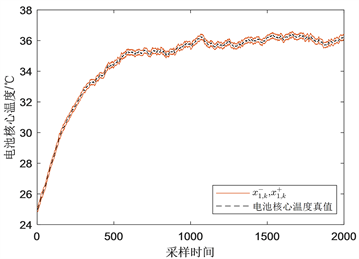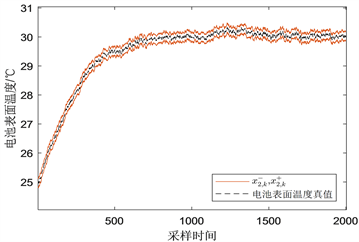﻿ 基于滤波的锂电池电热耦合特性建模

基于滤波的锂电池电热耦合特性建模Modeling of Electro-Thermal Coupled Characteristics of Li-Ion Battery Based on Filtering

Abstract: According to the heat generation mechanism, a thermal model of the Li-ion battery is constructed, combined with the second-order Thevenin equivalent circuit model of the Li-ion battery, and an electrothermal coupled model is constructed for the electrothermal coupled characteristics of Li-ion batteries. Taking into account the model error and the measurement noise in the measurement process, a Zonotopic filter is designed to realize the modeling of the electrothermal coupling char-acteristics of the Li-ion battery.

1. 引言

2. 模型建立Figure 1. The schematic diagram of the second-order Thevenin model

$\begin{array}{l}U={U}_{oc}-{R}_{o}I-{U}_{1}-{U}_{2}\\ \left\{\begin{array}{l}{\stackrel{˙}{U}}_{1}=-\frac{1}{{R}_{1}{C}_{p1}}{U}_{1}+\frac{1}{{C}_{p1}}I\\ {\stackrel{˙}{U}}_{2}=-\frac{1}{{R}_{2}{C}_{p2}}{U}_{2}+\frac{1}{{C}_{p2}}I\end{array}\end{array}$ (1)Figure 2. Two-state radial thermal model

$\left\{\begin{array}{l}{C}_{c}{\stackrel{˙}{T}}_{c}={Q}_{gen}+\frac{{T}_{s}-{T}_{c}}{{R}_{c}}\hfill \\ {C}_{s}{\stackrel{˙}{T}}_{s}=\frac{{T}_{e}-{T}_{s}}{{R}_{u}}-\frac{{T}_{s}-{T}_{c}}{{R}_{c}}\hfill \end{array}$ (2)

${Q}_{gen}=I\left({U}_{oc}-U\right)+IT\frac{\partial {U}_{oc}}{\partial T}$ (3)

$\left\{\begin{array}{l}{\stackrel{˙}{U}}_{1}=-\frac{1}{{R}_{1}{C}_{p1}}{U}_{1}+\frac{1}{{C}_{p1}}I\\ {\stackrel{˙}{U}}_{2}=-\frac{1}{{R}_{2}{C}_{p2}}{U}_{2}+\frac{1}{{C}_{p2}}I\end{array}$ (4)

$\begin{array}{l}{\stackrel{˙}{T}}_{c}=-\frac{{T}_{c}}{{R}_{c}{C}_{c}}+\frac{{T}_{s}}{{R}_{c}{C}_{c}}+\frac{I\left({U}_{1}+{U}_{2}+{R}_{o}I\right)}{{C}_{c}}\\ {\stackrel{˙}{T}}_{s}=-{T}_{s}\left(\frac{1}{{R}_{c}{C}_{s}}+\frac{1}{{R}_{u}{C}_{s}}\right)+\frac{{T}_{c}}{{R}_{c}{C}_{s}}+\frac{{T}_{e}}{{R}_{u}{C}_{s}}\end{array}$ (5)

$\left\{\begin{array}{l}\left[\begin{array}{c}{T}_{c,k+1}\\ {T}_{s,k+1}\end{array}\right]=\left[\begin{array}{cc}1-\frac{\text{Δ}t}{{R}_{c}{C}_{c}}& \frac{\text{Δ}t}{{R}_{c}{C}_{c}}\\ \frac{\text{Δ}t}{{R}_{c}{C}_{s}}& 1-\frac{\text{Δ}t}{{R}_{c}{C}_{s}}-\frac{\text{Δ}t}{{R}_{u}{C}_{s}}\end{array}\right]\left[\begin{array}{c}{T}_{c,k}\\ {T}_{s,k}\end{array}\right]+\left[\begin{array}{cc}\frac{\text{Δ}t}{{C}_{c}}& 0\\ 0& \frac{\text{Δ}t}{{R}_{u}{C}_{s}}\end{array}\right]\left[\begin{array}{c}{Q}_{gen,k}\\ {T}_{e,k}\end{array}\right]+\left[\begin{array}{cc}1& 0\\ 0& 1\end{array}\right]{w}_{k}\\ \left[\begin{array}{c}{\stackrel{¯}{T}}_{c,k}\\ {\stackrel{¯}{T}}_{s,k}\end{array}\right]=\left[\begin{array}{cc}1& 0\\ 0& 1\end{array}\right]\left[\begin{array}{c}{T}_{c,k}\\ {T}_{s,k}\end{array}\right]+\left[\begin{array}{cc}1& 0\\ 0& 1\end{array}\right]{v}_{k}\end{array}$ (6)

$\left[\begin{array}{cc}1-\frac{\text{Δ}t}{{R}_{c}{C}_{c}}& \frac{\text{Δ}t}{{R}_{c}{C}_{c}}\\ \frac{\text{Δ}t}{{R}_{c}{C}_{s}}& 1-\frac{\text{Δ}t}{{R}_{c}{C}_{s}}-\frac{\text{Δ}t}{{R}_{u}{C}_{s}}\end{array}\right]$ 表示状态空间矩阵； $\left[\begin{array}{cc}\frac{\text{Δ}t}{{C}_{c}}& 0\\ 0& \frac{\text{Δ}t}{{R}_{u}{C}_{s}}\end{array}\right]$ 表示输入矩阵。

3. 基于全对称多胞体滤波的电热耦合特性建模

s阶全对称多胞体 $\mathcal{Z}\subset {R}^{n}$ 是超立方体 ${B}^{s}={\left[-1,+1\right]}^{s}$ 的仿射变换 ，即：

${\mathcal{Z}}_{k}=p+\underset{i=1}{\overset{s}{\sum }}{\alpha }_{i}{g}_{i}={p}_{k}\oplus {G}_{k}{B}^{s}=〈{p}_{k},{G}_{k}〉$ (7)

$〈{p}_{1},{G}_{1}〉\oplus 〈{p}_{2},{G}_{2}〉=〈{p}_{1}+{p}_{2},\left[{G}_{1}\text{}{G}_{2}\right]〉$ (8)

$M\otimes 〈p,G〉=〈Mp,MG〉$ (9)

$\left\{\begin{array}{l}{x}_{k+1}=A{x}_{k}+B{u}_{k}+{D}_{1}{w}_{k}\\ {y}_{k}=C{x}_{k}+{D}_{2}{v}_{k}\end{array}$ (10)

${v}_{k}\in {ℝ}^{v}$ 分别表示系统未知但有界的扰动噪声和测量噪声，即 $|{w}_{k}|\le \stackrel{˜}{w}$，即 $|{v}_{k}|\le \stackrel{˜}{v}$

$\begin{array}{l}{x}_{0}\in \mathcal{X}=〈{p}_{0},{G}_{0}〉\\ {w}_{0}\in \mathcal{W}=〈0,W〉\\ {v}_{0}\in \mathcal{V}=〈0,V〉\end{array}$ (11)

(1) 预测步

${x}_{k}\in {\mathcal{Z}}_{k}={p}_{k}\oplus {G}_{k}{B}^{s}=〈{p}_{k},{G}_{k}〉$ (12)

${\stackrel{^}{\chi }}_{k+1}=〈{\stackrel{^}{p}}_{k+1},{\stackrel{^}{G}}_{k+1}〉$ (13)

$\begin{array}{l}{\stackrel{^}{p}}_{k+1}=A{p}_{k}+B{u}_{k}\\ {\stackrel{^}{G}}_{k+1}=\left[\begin{array}{cc}A{G}_{k}& {D}_{1}W\end{array}\right]\end{array}$ (14)

$\mathcal{S}=\left\{{x}_{k+1}\in {ℝ}^{n}:|C{x}_{k+1}-{y}_{k+1}|\le {D}_{2}\stackrel{˜}{v}\right\}$ (15)

(2) 更新步

$\begin{array}{l}p\left(\Lambda \right)=p+\Lambda \left(c-Kp\right)\\ G\left(\Lambda \right)=\left[\begin{array}{cc}\left({I}_{n}-\Lambda K\right)G& \Lambda \Delta \end{array}\right]\end{array}$ (16)

$\mathcal{Z}\cap \mathcal{S}\subseteq \mathcal{Z}\left(\Lambda \right)=〈p\left(\Lambda \right),G\left(\Lambda \right)〉$ (17)

${p}_{k+1}\left({L}_{k+1}\right)={\stackrel{^}{p}}_{k+1}+{L}_{k+1}\left({y}_{k+1}-C{\stackrel{^}{p}}_{k+1}\right)$ (18)

${G}_{k+1}\left({L}_{k+1}\right)=\left[\left(I-{L}_{k+1}C\right){\stackrel{^}{G}}_{k+1}\text{ }{L}_{k+1}{D}_{v}\right]$ (19)

${L}_{k+1}={\stackrel{^}{G}}_{k+1}{\stackrel{^}{G}}_{k+1}^{\text{T}}{C}^{\text{T}}×{\left(C{\stackrel{^}{G}}_{k+1}{\stackrel{^}{G}}_{k+1}^{\text{T}}{C}^{\text{T}}+{D}_{v}{D}_{v}^{\text{T}}\right)}^{-1}$ (20)

${x}_{k+1}\in {\chi }_{k+1}=〈{p}_{k+1},{G}_{k+1}〉$ (21)

${p}_{k+1}={\stackrel{^}{p}}_{k+1}+{L}_{k+1}\left({y}_{k+1}-C{\stackrel{^}{p}}_{k+1}\right)$ (22)

${G}_{k+1}=\left[\left(I-{L}_{k+1}C\right){\stackrel{^}{G}}_{k+1}\text{ }{L}_{k+1}{D}_{v}\right]$ (23)

${L}_{k+1}={\stackrel{^}{G}}_{k+1}{\stackrel{^}{G}}_{k+1}^{\text{T}}{C}^{\text{T}}×{\left(C{\stackrel{^}{G}}_{k+1}{\stackrel{^}{G}}_{k+1}^{\text{T}}{C}^{\text{T}}+{D}_{v}{D}_{v}^{\text{T}}\right)}^{-1}$ (24)

$\left\{\begin{array}{l}{x}_{k+1}^{+}\left(i\right)={p}_{k+1}\left(i\right)+\underset{j=1}{\overset{s}{\sum }}|{G}_{k+1}\left(i,j\right)|,\text{\hspace{0.17em}}i=1,\cdots ,n\hfill \\ {x}_{k+1}^{-}\left(i\right)={p}_{k+1}\left(i\right)-\underset{j=1}{\overset{s}{\sum }}|{G}_{k+1}\left(i,j\right)|,\text{\hspace{0.17em}}i=1,\cdots ,n\hfill \end{array}$ (25)

4. 仿真示例Table 1. Main parameters of electro-thermal coupled characteristics of Li-ion batteryFigure 3. Upper and lower bound estimates of core temperature in batteryFigure 4. Upper and lower bound estimates of surface temperature in battery

5. 结论

NOTES

*通讯作者。

 陈锦攀, 赵鸿, 钟国彬, 等. 锂电池储能系统专利技术发展态势[J]. 储能科学与技术, 2019, 8(3): 602-608.

 苏建彬. 动力电池热模型研究现状[J]. 汽车实用技术, 2019(20): 18-21.

 杨俊. 车用锂电池电化学建模、估计与老化研究[D]: [硕士学位论文]. 上海: 上海交通大学, 2019.

 Dey, S., Biron, Z.A., Tatipamula, A.S., et a1. (2016) Model-Based Real-Time Thermal Fault Diagnosis of Lithium-Ion Batteries. Control Engineering Practice, 56, 37-48.
https://doi.org/10.1016/j.conengprac.2016.08.002

 Scott, J.K., Findeisen, R., Braatz, R.D. and Raimon-do, D.M. (2014) Input Design for Guaranteed Fault Diagnosis Using Zonotopes. Automatica, 50, 1580-1589.

 徐兴, 王位, 陈龙. 基于GA的车用锂离子电池电化学模型参数辨识[J]. 汽车工程, 2017, 39(7): 813-821, 838.

 Chabane, S.B., Maniu, C.S., Alamo, T., et al. (2014) Sensor Fault Detection and Diagnosis Using Zonotopic Set-Memberbership Estimation. IEEE Mediterranean Conference on Control and Automation, Palermo, 16-19 June 2014, 261-266.

 汤文涛, 王振华, 王烨, 等. 基于未知输入集员滤波器的不确定系统故障诊断[J]. 自动化学报, 2018, 44(9): 1717-1724.

Top